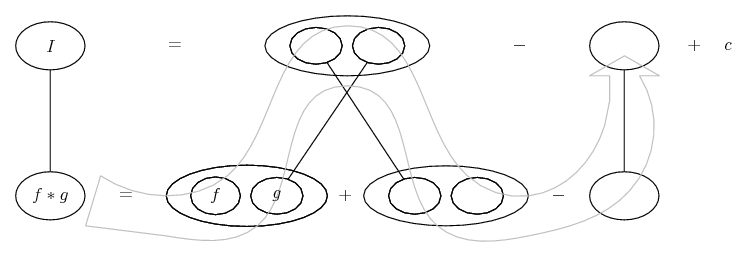# Thread: Finding the area under a curve using integration of trig functions.

1. ## Finding the area under a curve using integration of trig functions.

Hello!!

I had an integration exam today and I'm desperate to know if I answered this question correctly. As follows....

Find the area under the curve when Y= 5xsin(2x) when x=0, x=PI/2

I got 452, which I'm 99% sure is wrong but you never know!!

Thanks for your help and time in advance.

Chris. x

2. ## Re: Finding the area under a curve using integration of trig functions.

Bad luck. int 5x sin(2x) - Wolfram|Alpha

Just in case a picture helps...... where (key in spoiler) ...

Spoiler:... is the product rule. Straight continuous lines differentiate downwards (integrate up) with respect to x. And,... is lazy integration by parts, doing without u and v.

_________________________________________

Don't integrate - balloontegrate!

Balloon Calculus; standard integrals, derivatives and methods

Balloon Calculus Drawing with LaTeX and Asymptote!

3. ## Re: Finding the area under a curve using integration of trig functions.

Hello, Purn!

Find the area under the curve: .$\displaystyle y \:=\: 5x\sin(2x)\:\text{ for }x \in [0,\tfrac{\pi}{2}]$

You need to integrate by parts . . .

We have: .$\displaystyle I \;=\;\int 5x\sin(2x)\,dx$

.$\displaystyle \begin{Bmatrix}u &=& 5x && dv &=& \sin2x\,dx \\ du &=& 5\,dx && v &=& \text{-}\frac{1}{2}\cos(2x) \end{Bmatrix}$

$\displaystyle \text{Then: }\:I \;=\;\text{-}\tfrac{5}{2}x\cos(2x) + \tfrac{5}{2}\int\cos(2x)\,dx$

n . . . . . $\displaystyle =\;\text{-}\tfrac{5}{2}x\cos(2x) + \tfrac{5}{4}\sin(2x)\,\bigg]^{\frac{\pi}{2}}_0$

n . . . . . $\displaystyle =\;\bigg[\text{-}\tfrac{5}{2}\left(\tfrac{\pi}{2}\right)\cos\pi + \tfrac{5}{4}\sin\pi\bigg] - \bigg[\text{-}\tfrac{5}{2}(0)\cos0 + \tfrac{5}{4}\sin0\bigg]$

n . . . . . $\displaystyle = \; \text{-}\tfrac{5}{4}\pi(\text{-}1)$

n . . . . . $\displaystyle =\;\frac{5\pi}{4}$

4. ## Re: Finding the area under a curve using integration of trig functions.Originally Posted by PurnHello!!

I had an integration exam today and I'm desperate to know if I answered this question correctly. As follows....

Find the area under the curve when Y= 5xsin(2x) when x=0, x=PI/2

I got 452, which I'm 99% sure is wrong but you never know!!

Thanks for your help and time in advance.

Chris. x
You might, by the way, have notice that since sine is never larger than 1, "5x sin(x/2)", between x= 0 and $\displaystyle x= \pi/2$, cannot be larger than $\displaystyle 5(\pi/2)$ and so its integral cannot be larger than $\displaystyle 5(\pi/x)^2$ which is about 12.33. I can't imagine how you got "452"!

,

,

,

,

### Find the area under the curve in trig fcns

Click on a term to search for related topics.

#### Search Tags

area, curve, finding, functions, integration, trig